## Calculation of structure factors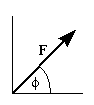We know by now how to calculate the individual atomic scattering factors f(j) which we need in the calculation of the structure factors :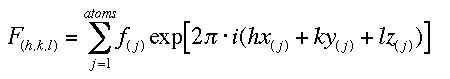(1)

The structure factors F(hkl) are directly related to the Intensity I (hkl) of the corresponding reflection h,k,l :(2)

LP is a combined geometry and polarization factor which depends on the particular experimental setup. It is usually accounted for in the early stages of data processing. A is an absorption correction factor which may or may not be applied.

Note again that we cannot calculate F from the intensity. Only the scalar magnitude of F, |F|, and not the complex scattering factor F itself is available to us directly from the experiment. This is the root cause of the phase problem. At this point, however, we do not want to calculate structure from diffraction data, we want to see how different atoms and different arrangements of atoms in the unit cell influence the structure factor. We will also examine how anomalous contributions to the atomic scattering factor result in the breakdown of Friedel's law.

# Exercises

The following program lets you calculate structure factors for a simple one-dimensional structure. You can select cell dimensions, two atoms, their type, position, and B-factor, and the X-ray anode (wavelength) used in the experiment. NOTE : you will have to select a unique file name in order to use your structure factors for a subsequent Fourier Synthesis !
The actual calculation of the structure factor uses Euler's formula for the complex exponential function (it can be derived easily by comparing the Taylor expansions of exp, sin, and cos):(3)

We can write the phase contribution of the individual atom iand using (3)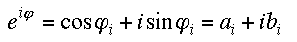we can rewrite formula (1) for F as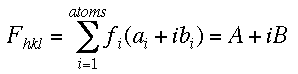The phase angle of each reflection is then given as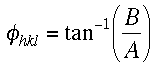(5)

We can already see that for a centrosymmetric structure, all coefficients b (and B) will be zero out as sin(x) = -sin(-x), and the phase angles thus limited to 0 or 180 degrees, depending on the sign of A. We will also see that this is strictly true only for zero anomalous contributions to f, and that in the centrosymmetric case Friedel's law (|F(hkl)| = |F(-hkl)|) still holds despite the anomalous contribution (see the use of centric zones/reflections in the MAD tutorial). The effect of anomalous scattering is zero in this case.

• A useful way to visualize Fs is to present them as vectors in the complex plane using a vector or Argand diagram. Take a look and the formulas above will become quite clear.

Exercises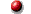First, submit the program with the default values (generic non-centrosymmetric), save the output and have a look at the listing :You should be already familiar with the table of the Cromer-Mann coefficients from the tutorial on atomic scattering factors. In addition, there are now extra entries for the anomalous contributions f' and f". Note that they are really small (a few hundredth of an electron for light protein atoms at Cu wavelength), but they do exist.

Next, a table lists the atoms, positions, B-factors and whether anomalous dispersion is used in the following calculation. The main part then is a listing of the calculated reflections which contains the index of the reflection (h), the diffraction angle (2theta), the resolution or lattice plane distance (d), the intensity of the reflection (I), the magnitude of the structure factor (|F(h)|), and the real part (A), the imaginary part (B) and the phase (Phi) of the structure factor.

Note that both the values for F and the phases have no obvious restrictions. You see that Friedel's law holds, |F(-h)| is equal to |F(h)|, the phases of a pair are on opposite sites of zero. The trend of reflections to get weaker at higher resolution is also obvious; we have used real scattering factors and B-values and not theoretical point atoms. Note also that the value for F(0), which is in the transmitted beam path and not observable, is the sum of all electrons in the unit cell.

###Exercise 1 : B-factors and atom types

• Using the defaults, set both B-factors to 50, then to 0. What happens?
• Reload the defaults. Make the second atom a heavy atom. What happens?
• What does the value for F(0) represent? Check!

###Exercise 2 : Unit cell size and resolution

• Using the defaults, reduce/increase the cell size. What happens? What is the d-spacing of the first reflection?
• Reload the defaults, 20 A cell. Reduce the resolution to 3.5 A. What happens? Good or very bad?
• At what resolution would you observe reflections at around 90 deg 2theta using Cu radiation? What about their relative intensity? How could you increase their intensity? Hint: click here.
• What happens to the diffraction angles if we use a Mo tube? Why? Can we cheat and get more reflections at the same resolution?

###Exercise 3 : Atom positions, symmetry and centered cell

• Using the defaults, make both atoms the same and set x1 to 0.2, x2 to 0.8. What happened to the phases?
• Change one atom to a different kind. What happened to the phases now? Does the Friedel law still hold?
• Now make both atoms the same again, but set x1 to 0.3, and x2 to 0.8. What happened now? Does something look strange? Why?

###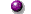Exercise 4 : Anomalous dispersion and the breakdown of Friedel's law

• Reload the default input, but click the anomalous scattering checkbox to YES. Does something happen? Is the effect significant?
• Now try other atoms, S, Se. Choose Fe. How big does the effect become? Why is it so strong for Fe? Would you want to merge the Friedel pairs in this case?
• Set both atoms to the same anomalous scatterer (e.g., 2 Fe or 2 Se atoms). What happens as far as Friedel's law is concerned? Any suggestions why? Hint : draw a vector diagram of the structure factors.
• What can the effect of anomalous scattering be used for?

You understand now how the unit cell contents determines phases and magnitude of structure factors. You should now proceed to the next step, namely to learn how to calculate electron density from structure factors of our simple models by means of Fourier transformations. You can click here to go directly to the Fourier program.Back to X-ray Tutorial Index
This World Wide Web site conceived and maintained by Bernhard Rupp.
Last revised Dezember 27, 2009 01:40